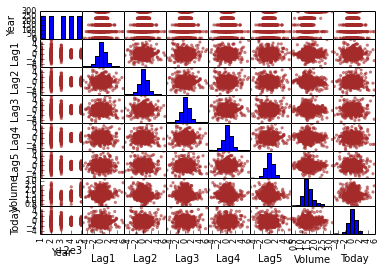Chapter 4: Classification¶

In :
from __future__ import division
import pandas as pd
import numpy as np
import matplotlib.pyplot as plt
from sklearn.linear_model import LogisticRegression
from sklearn.lda import LDA
from sklearn.neighbors import KNeighborsClassifier
from sklearn.metrics import confusion_matrix
from sklearn.metrics import accuracy_score
%matplotlib inline
In :
Out:
Year Lag1 Lag2 Lag3 Lag4 Lag5 Volume Today Direction
0 2001 0.381 -0.192 -2.624 -1.055 5.010 1.1913 0.959 Up
1 2001 0.959 0.381 -0.192 -2.624 -1.055 1.2965 1.032 Up
2 2001 1.032 0.959 0.381 -0.192 -2.624 1.4112 -0.623 Down
3 2001 -0.623 1.032 0.959 0.381 -0.192 1.2760 0.614 Up
4 2001 0.614 -0.623 1.032 0.959 0.381 1.2057 0.213 Up

5 rows × 9 columns

In :
# equivalent to the R pairs(df) command.
axes = pd.tools.plotting.scatter_matrix(smarket_df, color="brown")Logistic Regression¶

According to this blog post, Scikit-Learn's LogisticRegression has some significant differences from R's glm - to reproduce R's glm, one should use statsmodels.api.GLM. But my preference is to work with Scikit-Learn because of its greater breadth, so unless there is something that is only available from statsmodels, I am going to use Scikit-Learn. The numbers below are not identical but close.

In :
X = smarket_df[smarket_df.columns[1:-2]]
y = pd.factorize(smarket_df["Direction"])
clf = LogisticRegression()
clf.fit(X, y)
(clf.intercept_, clf.coef_)
Out:
(array([ 0.11454962]),
array([[ 0.07279035,  0.04229102, -0.010958  , -0.00921799, -0.01023031,
-0.12793931]]))
In :
# return probability for each class. R's predict() only returns the probability of the first
# class, so we do the same.
probs = clf.predict_proba(X)
[prob for prob in probs[0:5]]
Out:
[0.50775595757199166,
0.48208765404825193,
0.48147907945142998,
0.51561031224141374,
0.51134987027174317]
In :
ypreds = ["Up" if prob > 0.5 else "Down" for prob in probs]
ypreds[0:5]
Out:
['Up', 'Down', 'Down', 'Up', 'Up']
In :
# R uses table() to tabulate the confusion matrix below
yacts = [str(x) for x in smarket_df["Direction"].values]
confusion_matrix(yacts, ypreds)
Out:
array([[143, 459],
[135, 513]])
In :
accuracy_score(yacts, ypreds)
Out:
0.52480000000000004

Make Training and Test Set¶

In :
# Split dataset into training and test sets
smarket_train_df = smarket_df[smarket_df["Year"] < 2005]
smarket_test_df = smarket_df[smarket_df["Year"] >= 2005]
# train Logistic Regression model with training data
clf2 = LogisticRegression()
Xtrain = smarket_train_df[smarket_df.columns[1:-2]]
ytrain = pd.factorize(smarket_train_df["Direction"])
clf2.fit(Xtrain, ytrain)
# test model with test data
Xtest = smarket_test_df[smarket_df.columns[1:-2]]
ytest = pd.factorize(smarket_test_df["Direction"])
ypred = clf2.predict(Xtest)
# calculate confusion matrix and accuracy
confusion_matrix(ytest, ypred)
Out:
array([[40, 71],
[52, 89]])
In :
accuracy_score(ytest, ypred)
Out:
0.51190476190476186

Fit smaller model¶

In :
clf3 = LogisticRegression()
Xtrain = smarket_train_df[smarket_df.columns[1:3]]
Xtest = smarket_test_df[smarket_df.columns[1:3]]
clf3.fit(Xtrain, ytrain)
ypred = clf3.predict(Xtest)
confusion_matrix(ytest, ypred)
Out:
array([[ 76,  35],
[106,  35]])
In :
accuracy_score(ytest, ypred)
Out:
0.44047619047619047

Linear Discriminant Analysis¶

In :
clf4 = LDA()
clf4.fit(Xtrain, ytrain)
ypred = clf4.predict(Xtest)
ypred[0:5]
Out:
array([0, 0, 0, 0, 0])
In :
confusion_matrix(ytest, ypred)
Out:
array([[ 76,  35],
[106,  35]])
In :
accuracy_score(ytest, ypred)
Out:
0.44047619047619047

K-Nearest Neighbors¶

In :
clf5 = KNeighborsClassifier()
clf5.fit(Xtrain, ytrain)
ypred = clf5.predict(Xtest)
confusion_matrix(ytest, ypred)
Out:
array([[71, 40],
[82, 59]])
In :
accuracy_score(ytest, ypred)
Out:
0.51587301587301593